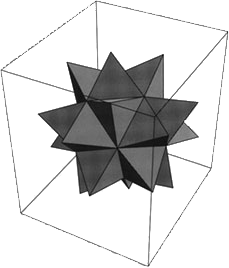the earliest Mathematica logo

In the earliest Mathematica documentation, including the development pages visible in the scrapbook on the Wolfram website, an extremely early version of their evolving polyhedral logo is visible. It looks like this:The image was, at the time, created with the following code:
``` In[]:= Needs["Graphics`Polyhedra`"]```

``` ```

```In[]:= Show[Graphics3D[Stellate[Icosahedron[]]]] ```

This code would have worked unmodified for many releases of the software, through version 5 I suspect. Nowadays, however, to get the same output we would have to refer to the new library “PolyhedronOperations”, and use a variety of new options to shut off the otherwise automatic multi-coloration of the figure.

``` In[]:= Needs["PolyhedronOperations`"]```

``` ```

```In[]:= Graphics3D[{Opacity, Glow[], FaceForm[Gray], Stellate[PolyhedronData["Icosahedron", "Faces"]]}, Lighting -> "Neutral"] ```

Mathematica in Finance and Economics conference, 4 August 2010

Wolfram is hosting a conference about the use of Mathematica in trading, financial modeling and simulation, data analysis and reporting, and other topics. In Chicago, unfortunately.

IRR and NPV in Mathematica

If you work in finance, you probably compute net present values (NPVs) and internal rates of return (IRRs) from time to time. As of version 7, Mathematica does not have built-in functions for these. There are add-on libraries that one might buy but it seems wrong to have to pay for something that is both so simple and so important. I have tried to convince Wolfram to add functions for computing these to the standard libraries, but thus far without success. So I will provide sample code here for anybody looking to do these things in Mathematica.

Generally when working with financial data series, I prefer to use data objects that have an identifying header rather than just raw data. In the long run, it makes life much simpler. In a later post I will explain this in more detail and give examples. For this post, I will not use that technique, and will show how to complete the calculations of NPV and IRR in Mathematica for a simple list of {date/datum} pairs.

First, since we are going to be using Mathematica’s date functions, and my sample dates are in “Month/Day/Year” format, we had better shut off some warnings:

`Off[AbsoluteTime::ambig]`

Now a function that takes a list of dates and a fixed annual discount rate, and returns a discount factor for each date.

``` sternDiscountFactors[dateList_, r_] := Module[{absDates, firstDate}, absDates = Map[AbsoluteTime[#] &, dateList]; firstDate = Min[absDates]; Transpose[{dateList, Map[1/(1 + r)^DateDifference[firstDate, #, "Year"][] &, absDates]}]] ```

For an example of how this works, run
`In[]:= sternDiscountFactors[{"1/1/2001", "1/1/2002", "1/1/2005", "1/10/2005"}, .05]`

``` ```

`Out[]= {{"1/1/2001", 1.}, {"1/1/2002", 0.952381}, {"1/1/2005", 0.822702}, {"1/10/2005", 0.821713}}`

Then we need a function for computing the net present value of a cash flow, given a fixed annual discount rate. Basically, it takes the dot product of vectors representing the discount factor on each date and the net payment on that date.

``` sternNetPV[dateAndCashFlowList_, r_] := Module[{cashflows, discountfactors}, cashflows = Transpose[dateAndCashFlowList][]; discountfactors = Transpose[sternDiscountFactors[Transpose[dateAndCashFlowList][], r]][]; cashflows.discountfactors] ```

Here is some sample data that we will use to test the NPV function and, in a minute, the IRR function:

`testFlow = {{"1/1/2001", -1}, {"1/1/2002", .3}, {"1/1/2005", .4}, {"1/10/2005", .5}};`

You can then test the NPV function with
`In[]:= sternNetPV[testFlow, .05]`

``` ```

`Out[]= 0.0256519`

Computing the internal rate of return is now simple:

```sternIrr[dateAndCashFlowList_] := Module[{eqn, r}, eqn = sternNetPV[dateAndCashFlowList, r] == 0; FindRoot[eqn, {r, .1}]]```

and this can be tested with
`In[]:= sternIrr[testFlow]`

``` ```

`Out[]={r\$3479 -> 0.0584316}`

Aquí tiene. In real world applications, one would not typically rely on a fixed annual discount rate, and the NPV function would in most important contexts use a yield curve instead.